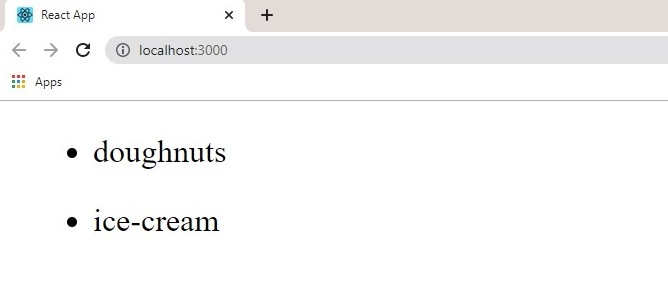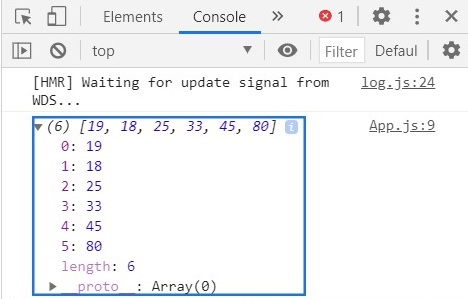# React Lists and Keys

Keys help React identify items in the DOM, which items have changed, been added and removed. Keys should be given to arrays when they perform array methods such as `map()`, `reduce()`, `filter(), find()` etc.

## Map()

For example, in App.js, write the following:

``````import React from "react";

const App = () => {
const numbers = [1, 2, 3, 4];
const squares = numbers.map((number) => (
<li key={number.toString}>{number * number}</li>
));
return (
<div>
<ul>{squares}</ul>
</div>
);
};

export default App;``````

A variable ‘`numbers`’ was assigned an array, we desire to render the square of the numbers in the browser. So, we use `map()` to go through each number in the array then return a `<li>` element containing squares of each number in the array. Then we store this value in a variable ‘squares’ and then render it to a `<ul>` tag to be displayed.

Notice the attribute ‘`key`’ in the list tag, this is necessary as it allows React to correctly identify each number in the array.

Another example might be to map through an array containing objects with a name and an id,

`` [{id: 1001, name: 'ice-cream'}, {id: 1002, name: 'doughnut'}]``

Products.js

``````import React from "react";

const Products = () => {
const productArray = [{id: 1001, name: 'doughnuts'}, {id:1002, name: 'ice-cream'}];
const productList = productArray.map((items) => (

<ul>
<li key={items.id.toString()}>{items.name}</li>
</ul>
));
return (
<div>
{productList}
</div>
);
};

export default Products;``````

### Result:## Reduce()

The reduce function takes in two arguments, an accumulator and current value. It basically reduces an array to a single value, can be good to add up an array of numbers, e.g. prices in a cart.

Products.js

``````import React from "react";

const Products = () => {
const prices = [19.99, 179.99, 100, 89.99];
const Total = prices.reduce((accumulator, current) => (
accumulator + current
));
return (
<div>
{Total}
</div>
);
};

export default Products;``````

## Filter()

This javascript function filters an array based on a set specification. For example, to filter ages above 18, we can have the following code:

Vote.js:

``````import React from "react";

const Vote = () => {
const ages = [19, 13, 18, 25, 33, 45, 7, 80];
const canVote = ages.filter((age) => age >= 18);
console.log(canVote);
return (
<div>
<></>
</div>
);
};

export default Vote;
``````

### Result:## Find()

This function takes in a function that checks for an element in an array and returns the first match.

Vote.js:

``````import React from "react";

const Vote = () => {
const ages = [19, 13, 18, 25, 18, 33, 45, 7, 80];
const canVote = ages.find((age) => (age > 17));
console.log(canVote);
return (
<div>
<></>
</div>
);
};

export default Vote;
``````

the code above will result in 19 as 19 is the first match to be greater than 17.